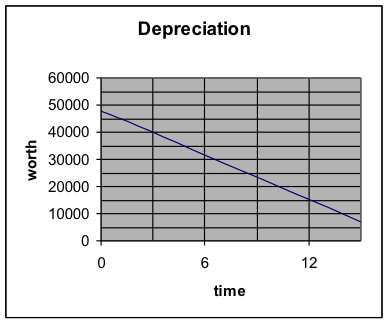## H1.03: Example 2

Example 2: Ajax Manufacturing bought a machine for $48,000. It is expected to last 15 years and, at the end of that time, have a salvage value of$7,000. Set up a linear depreciation model for this machine and find the worth at the end of 10 years.

Solution:

1. What are the two variables and their units?

Ans. Time and worth. Time is measured in years and worth in dollars.

2. What will we predict? (Make it y.)  What are some points?

Ans. We will predict worth. So y = worth, which must always be above $7000. Then x = years and x will go from 0 to 15. At zero years, the worth is 48000. So x = 0 and y = 48000. Use (0, 48000) At 15 years, the worth is 7000. So x = 15 and y = 7000. Use (15, 7000) 3. Is a linear model appropriate? Yes, because the problem asks for linear depreciation. 4. Slope Find the slope: m = rise / run Let (0,48000) be the first point and (15,7000) be the second point. $m=\frac{{{y}_{2}}-{{y}_{1}}}{{{x}_{2}}-{{x}_{1}}}=\frac{48000-7000}{0-15}=\frac{41000}{-15}=-2733.333$ Interpret the slope, using the units of the numbers in the problem: (As x increases by 1, y increases by m.) For each year, the worth increases by -2733.33. But, of course, increasing by a negative amount means decreasing. So, for each year, the worth decreases by$2733.33.

5. Write the formula of the linear relationship:

(Choose either point and use the point-slope form of the line. Then simplify it to the slope-intercept form of the line.)

Choose (0, 48000) for the point and use the slope of -2733.333.

\begin{align}&y-{{y}_{0}}=m(x-{{x}_{0}})\\&y-48000=-2733.333(x-0)\\&y-48000=-2733.333x\\&y=-2733.333x+48000\\\end{align}

6. Interpret the y-intercept.

(The value of y is b when .)

At the beginning of the process, when time=0, the worth is 48,000. 7. Use the equation to make the requested prediction. Write the result in a sentence, with units. At 10 years, x = 10: \begin{align}&y=-2733.333x+48000\\&y=-2733.333(10)+48000\\&y=-27333.33+48000\\&y=20666.67\end{align} So the worth of the machine at 10 years is20,666.67.

8. Make a graph and use it to check the prediction.

 time worth 0 48000 15 7000

By hand, we plot these points, draw the line, and use it to estimate the answer to the question.Notice that the graph slants down, which means the slope is negative. And notice that you did get a negative slope using the formulas. That’s good!

On the graph, look for time=10, and see that the worth is about \$20,000.

That is consistent with the value obtained using the formula.

The prediction is reasonable.